Math Concepts

# Analytical Reasoning

11th Sep '205 views6 mins read

 1 What is Analytical Reasoning? 2 Real Life Example 3 Figure Type Problems 3 Try Out Following To Check Your Understanding

September 14, 2020,

In this blog the readers will know about Analytical Reasoning and How to solve some specific types of Analytical Reasoning Questions. After reading the blog the reader will be able to find the number of straight lines, squares and geometrical shapes to make some specific figures. Not only this, Analytical reasoning can be applied in many other types of problems.

## What is Analytical Reasoning?

Analytical Reasoning refers to the ability to look at qualitative and quantitative information. Analytical Reasoning involves deductive reasoning, which means the problem may be solved with no specialized knowledge. The deduction is made on the basis of the information given in the question. It does not require any specific knowledge other than understanding of some specific words.

Analytical Reasoning is a part of many competitive tests. Through Analytical Reasoning a candidate’s comprehension skills and ability to identify key information, apply logic and find patterns is checked in most of these tests. There are different strategies for approaching the solution of analytical reasoning questions, so you should experiment to find which takes minimum time and works for you.

To better understand the topic, let’s work together on some examples.

## Real Life ExampleExample :

Given information:-

Seven tabla students—A, B, C, D, E, F, and G have to perform, and their instructor has to decide the order in which they will perform. Each student will play exactly one piece of solo. For deciding the order of performance, the instructor has to observe the following restrictions:

1. E cannot play first or second.
2. D cannot play until E has played.
3. Neither A nor F can play seventh.
4. Either F or G must play immediately after D plays.
5. C must play either immediately after or immediately before B plays.

Question: If C plays first, which one of the following must be true?

1. A plays sixth.
2. E plays third.
3. G plays seventh.
4. A plays immediately after F.
5. E plays immediately after D.

Explanation of solution

This problem deals with a specific order in which tabla students will perform. One way to approach this problem is to write down the order of seven students from left to right, as illustrated below. Student C is shown in the first slot, as specified in question that “C plays first”:

1     2     3     4     5     6     7

C

Depending on the given conditions we keep on filling all seven positions. The condition that “C must play either immediately after or immediately before B plays” indicates that B must occupy the second position in the schedule. This is shown below:

1     2     3     4     5     6     7

C     B

Now the question asks us what must be true, so we can eliminate incorrect responses. Response (1) is incorrect because the statement that “A plays sixth” is not necessarily true, rather it can be placed at any position. One such schedule can be with A playing third, is shown below:

1     2     3     4     5     6     7

C     B    A    E    D     F    G

This schedule can be derived as follows:

With C, B, and A at the first three positions, there are four positions left for D, E, F, and G.

D must come after E—because of the condition that “D cannot play until E has played”—so if E is at fourth position then D is fifth and the condition will be met.

This leaves two possible slots for F and G. F cannot play seventh because of the condition that “Neither A nor F can play seventh.” So when F is sixth and G is seventh.

We verify that this schedule meets the conditions of the problem, including the one that “Either F or G must play immediately after D plays.”

The schedule shown above also demonstrates that response (2) is incorrect. In it, E plays fourth, so it is not correct that “E plays third” is true.

Response (3), “G plays seventh,” is the only correct response.

Similarly we can easily check that other options are also incorrect.

Basically Analytical Reasoning means to gather and analyze information in order to solve problems.

## Figure Type Problems

Minimum number of straight lines required to draw the figure.

 Example 1 :

Find the minimum number of straight lines required to make the given figure.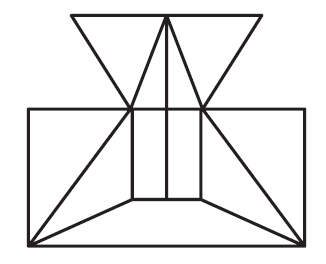(A) 16                (B) 17                (C) 19                (D) 21

Explanation of solution

Start by counting horizontal lines – 4, then vertical lines – 5 and in the end slanting lines – 8. Hence total lines become 17.

 Correct option is B

Counting the number of squares, triangles, and rectangles in the given figure.

 Example 2:

Find the number of squares in each of the given figures.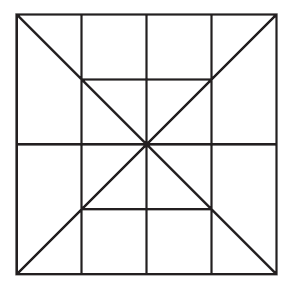(A) 18               (B) 15               (C) 19              (D) 16

Explanation of solution

To deduce the answer we start by counting single squares, which are 8 in our figure. Then squares of second larger dimension, which arte 7. Ans in the end largest square is 1. Total of all of them is 16

 Correct option is D

Counting the number of triangles, squares and rectangles in the given figure.

 Example 3:

Find the number of triangles in the given figure.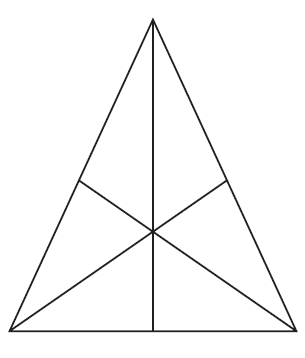(A) 16               (B) 13               (C) 9               (D) 7

Explanation of solution

Consider a small triangle as a single unit. First start by taking single unit triangles – 6, two unit triangles – 3, triangles with three unit triangles – 6, and finally a triangle with six unit triangles – 1. This gives a total of 16.

 Correct option is A

Counting the number of squares, triangles, and rectangles in the given figure.

 Example 4:

Find the number of rectangles in the given figure.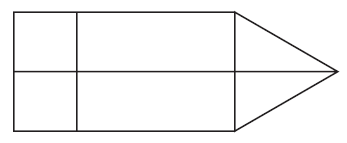(A) 4              (B) 5              (C) 7              (D) 8

Explanation of solution

In this figure there are rectangles with different dimensions. Start with the smallest triangle to the largest triangle. From the above two examples you can take an idea of count to judge that the number of rectangles in the above figure is 7.

 Correct option is C
 Example 5:

How many triangles and squares are there in the given figure?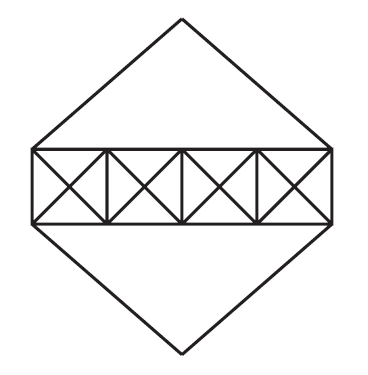(A) 36 triangles, 7 squares                           (B) 38 triangles, 9 squares

(C) 40 triangles, 7 squares                           (D) 42 triangles, 9 squares

Explanation of solution

Inside each square there are four small triangles with one unit and four triangles of two units each. So inside small squares there are 8 triangles. Multiplying with the number of squares we get 32 triangles. Then there are four unit triangles – 6 and last are two big triangles on top and bottom so that makes a total of 40 triangles. The seven squares (four big and three small) are clearly visible.

 Correct option is C
 Example 6:

Count the number of parallelograms in the given figure.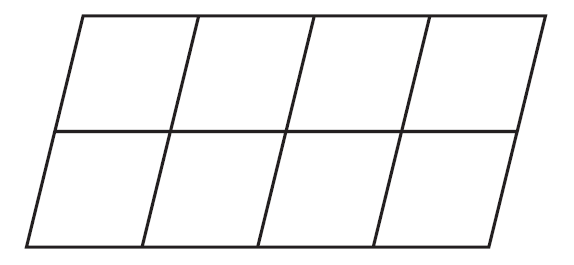(A) 30               (B) 24               (C) 25               (D) 27

Explanation of solution

Start by counting single unit parallelograms – 8, two units parallelograms are vertical (4) as well as horizontal (6), three units parallelograms are 6, four units parallelograms are also vertical (3) and horizontal (2). Then we have two parallelograms with six units and the largest one parallelogram with all units included. So we get a total of 30 parallelograms.

 Correct option is A
 Example 7:

How many semicircles are there in the given figure?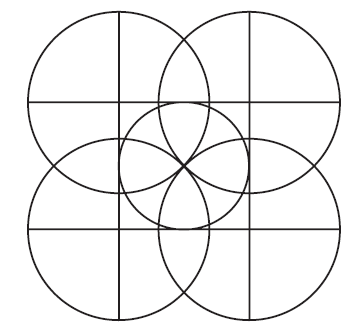(A) 8               (B) 12              (C) 16              (D) 20

Explanation of solution

Concentrating on the first circle in the extreme left corner, we can check that it can be divided in two ways to get four semicircles. Like that we have four circles so in total we have 16 semicircles. There is no other part of the circle which is divided as a semicircle.

 Correct option is C

How many pentagons are there in the given figure?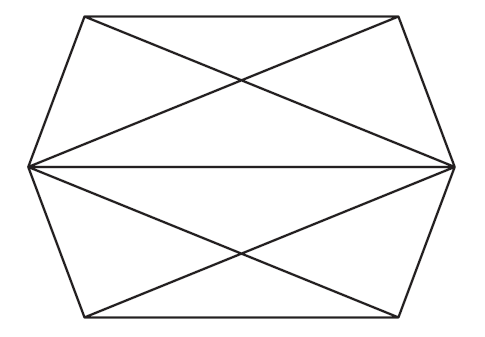(A) 2              (B) 3              (C) 4              (D) 6

Explanation of solution

If we start from each corner we can make one pentagon. This makes a total of 6 pentagons.

 Correct option is D

## Try Out Following To Check Your Understanding

1. For the following picture find the number of Triangles: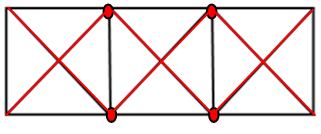2. Count the total number of Triangles in the following figure: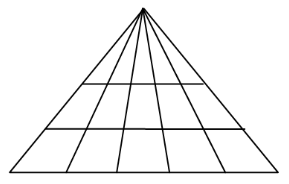I hope the blog has helped the reader to understand what is Analytical Reasoning. And how do we count the various options by Observing the figure carefully and counting them separately. Different examples cited will also help the reader to be able to combine figures to form one figure, counting each step by step and adding them to arrive at a solution. Little practice can make you proficient.

Related Articles
GIVE YOUR CHILD THE CUEMATH EDGE
Access Personalised Math learning through interactive worksheets, gamified concepts and grade-wise courses